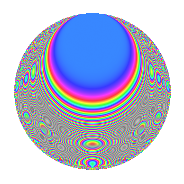# Properties

 Label 672.2.bdLevel 672 Weight 2 Character orbit bd Rep. character $$\chi_{672}(431,\cdot)$$ Character field $$\Q(\zeta_{6})$$ Dimension 56 Newform subspaces 1 Sturm bound 256 Trace bound 0

# Related objects

## Defining parameters

 Level: $$N$$ = $$672 = 2^{5} \cdot 3 \cdot 7$$ Weight: $$k$$ = $$2$$ Character orbit: $$[\chi]$$ = 672.bd (of order $$6$$ and degree $$2$$) Character conductor: $$\operatorname{cond}(\chi)$$ = $$168$$ Character field: $$\Q(\zeta_{6})$$ Newform subspaces: $$1$$ Sturm bound: $$256$$ Trace bound: $$0$$

## Dimensions

The following table gives the dimensions of various subspaces of $$M_{2}(672, [\chi])$$.

Total New Old
Modular forms 288 72 216
Cusp forms 224 56 168
Eisenstein series 64 16 48

## Trace form

 $$56q + 2q^{3} - 2q^{9} + O(q^{10})$$ $$56q + 2q^{3} - 2q^{9} + 4q^{19} - 16q^{25} + 8q^{27} - 14q^{33} + 16q^{43} - 16q^{49} + 34q^{51} + 4q^{57} + 36q^{67} + 4q^{73} - 10q^{81} - 72q^{91} - 32q^{97} + 44q^{99} + O(q^{100})$$

## Decomposition of $$S_{2}^{\mathrm{new}}(672, [\chi])$$ into newform subspaces

Label Dim. $$A$$ Field CM Traces $q$-expansion
$$a_2$$ $$a_3$$ $$a_5$$ $$a_7$$
672.2.bd.a $$56$$ $$5.366$$ None $$0$$ $$2$$ $$0$$ $$0$$

## Decomposition of $$S_{2}^{\mathrm{old}}(672, [\chi])$$ into lower level spaces

$$S_{2}^{\mathrm{old}}(672, [\chi]) \cong$$ $$S_{2}^{\mathrm{new}}(168, [\chi])$$$$^{\oplus 3}$$

## Hecke Characteristic Polynomials

There are no characteristic polynomials of Hecke operators in the database